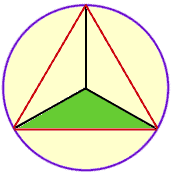Subject: Equilateral triangle in a circle Name: Michael Setlik Who is asking: Other Level: All Question: An equilateral triangle is drawn within a circle such that all three points of the triangle just touch the inside of the circle. Given the diameter of the cicle as six inches what is the length of the sides of the triangle? Hi Michael, Join each vertex of the triangle to the center of the circle to form three, congruent isosceles triangles. Condider one of these tringles, the one I have shaded green in the diagram.The angle in this triangle at the center of the circle has measure 360o/3 = 120o. The sum of the angles in any triangle is 180o hence each of the other angles in this triangle has measure (180o - 120o) = 30o. Thus the isosceles green triangle has two sides of length 3 inches, one angle of 120o and two angles of 30o each. You should now be able to find the length of the third side. Cheers,Harley Go to Math Central